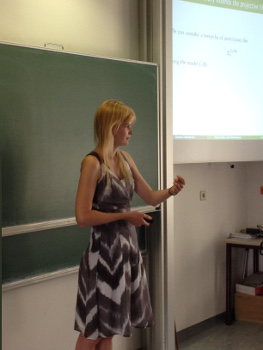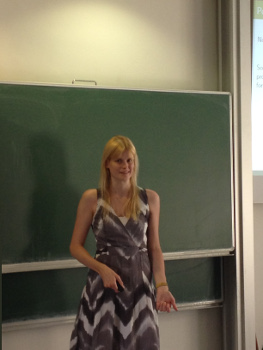On June 9th 2016 I gave a talk in the KGRC Research Seminar in Vienna.

Abstract: This talk will be an introduction to inner model theory the at the level of the $L(\mathbb{R})$-hierarchy. It will focus on results connecting inner model theory to the determinacy of certain games.

Mice are sufficiently iterable models of set theory. Martin and Steel showed in 1989 that the existence of finitely many Woodin cardinals with a measurable cardinal above them implies that projective determinacy holds. Neeman and Woodin proved a level-by-level connection between mice and projective determinacy. They showed that boldface $\boldsymbol\Pi^1_{n+1}$ determinacy is equivalent to the fact that the mouse $M_n^\sharp(x)$ exists and is $\omega_1$-iterable for all reals $x$.

Following this, we will consider pointclasses in the $L(\mathbb{R})$-hierarchy and show that determinacy for them implies the existence and $\omega_1$-iterability of certain hybrid mice with finitely many Woodin cardinals, which we call $M_k^{\Sigma, \sharp}$. These hybrid mice are like ordinary mice, but equipped with an iteration strategy for a mouse they are containing, which enables them to capture certain sets of reals. We will discuss what it means for a mouse to capture a set of reals and outline why hybrid mice fulfill this task. If time allows we will sketch a proof that determinacy for sets of reals in the $L(\mathbb{R})$-hierarchy implies the existence of hybrid mice.Many thanks to Richard for the pictures!